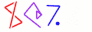Next You can't get there from here 9

```        sub total {
my (\$sizes, \$values, @items) = @_;
my \$total_size = 0;
my \$total_value = 0;
```
```          for my \$item (@items) {
\$total_size  += \$sizes->{\$item};
\$total_value += \$values->{\$item};
}
return (\$total_size, \$total_value);
}
```

 NextCopyright © 2005 M. J. Dominus